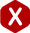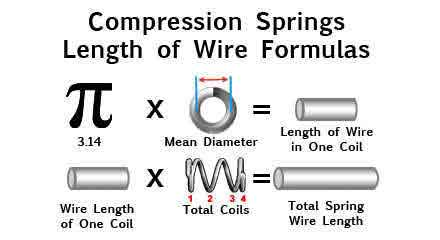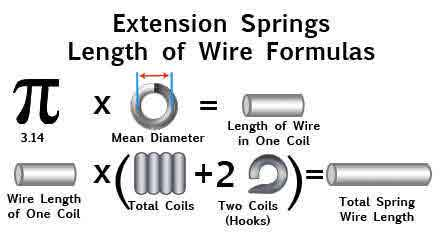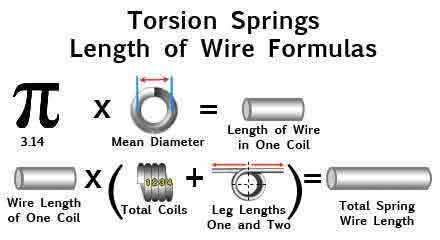<# Calculate Length of Coiled Spring Wire

#### Definition - The calculation or measurement of the length of the wire used to make a helical coil spring.

To calculate spring wire length per coil, you must subtract the wire diameter from the outer diameter in order to get mean diameter. Once you have calculated mean diameter, multiply it by pi (3.14); this will give you the length of wire per coil.

To calculate the length of wire to make a compression spring, multiply the length of wire per coil by the total number of coils. When it comes to extension springs you will have to add 2 coils to the active number of coils to include the hooks if the spring has cross over hooks or side hooks. For torsion springs, the process is also the same but you will add the leg lengths to the result. The formulas describing these calculations are provided below.

Calculate Compression Spring Wire Length In Inches:

3.14DN = Li

Calculate Extension Spring Wire Length In Inches:

3.14D (N + 2) = Li

Calculate Torsion Spring Wire Length In Inches:

3.14DN + LL1 + LL2 = Li

##### To calculate spring wire length in feet, use the following formulas.

Calculate Compression Spring Wire Length in Feet:

14DN ÷ 12 = Lf

Calculate Extension Spring Wire Length in Feet:

3.14D (N+2) ÷ 12 = Lf

Calculate Torsion Spring Wire Length in Feet:

(3.14DN + LL1 + LL2) ÷ 12 = Lf
##### Key:
• D = Mean Diameter
• N = Total Coils
• Li = Length (inches)
• Lf = Length (feet)
• LL1 = Leg Length 1
• LL2 = Leg Length 2